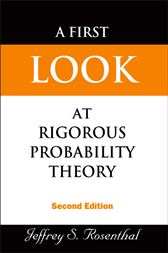# A First Look at Rigorous Probability Theory (2nd ed.)US\$26.00
US\$23.66
###### ISBNs
• 9789812703705
• 9789813101654

This textbook is an introduction to probability theory using measure theory. It is designed for graduate students in a variety of fields (mathematics, statistics, economics, management, finance, computer science, and engineering) who require a working knowledge of probability theory that is mathematically precise, but without excessive technicalities. The text provides complete proofs of all the essential introductory results. Nevertheless, the treatment is focused and accessible, with the measure theory and mathematical details presented in terms of intuitive probabilistic concepts, rather than as separate, imposing subjects. In this new edition, many exercises and small additional topics have been added and existing ones expanded. The text strikes an appropriate balance, rigorously developing probability theory while avoiding unnecessary detail.

• World Scientific Publishing Company; November 2006
• ISBN: 9789813101654
• Edition: 2